# Sine Function

The sine function is a periodic function which is very important in trigonometry.

The simplest way to understand the sine function is to use the unit circle. For a given angle measure $\theta$ , draw a unit circle on the coordinate plane and draw the angle centered at the origin, with one side as the positive  $x$ -axis. The  $y$ -coordinate of the point where the other side of the angle intersects the circle is $\mathrm{sin}\left(\theta \right)$ , and the  $x$ -coordinate is $\mathrm{cos}\left(\theta \right)$ .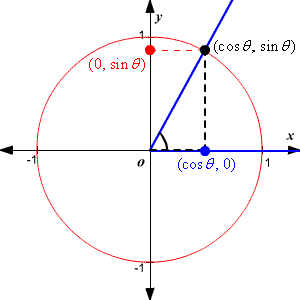There are a few sine values that should be memorized, based on $30°-60°-90°$ triangles and $45°-45°-90°$ triangles .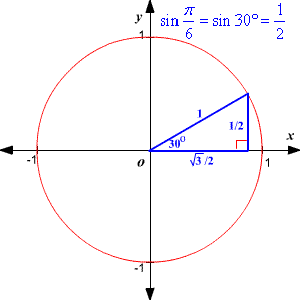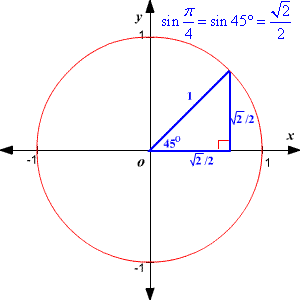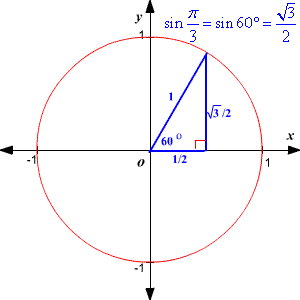Once you know these values, you can derive many other values for the sine function. Remember that $\mathrm{sin}\left(\theta \right)$ is positive in quadrants $\text{I}$ and $\text{II}$ and negative in quadrants $\text{III}$ and $\text{IV}$ .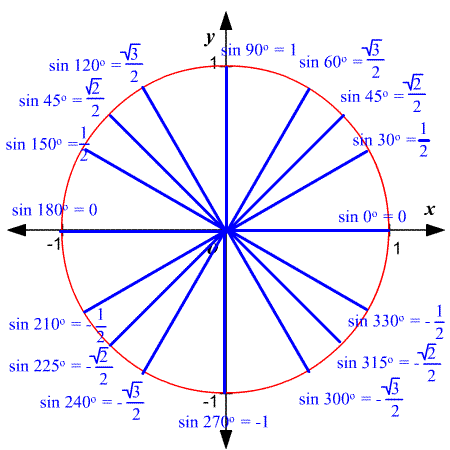You can plot these points on a coordinate plane to show part of the sine function, the part between $0$ and $\text{2}\pi$ .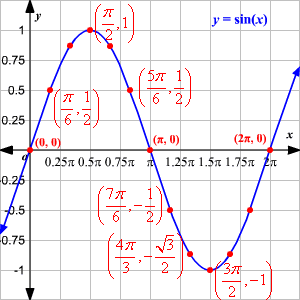For values of $\theta$ less than $0$ or greater than $\text{2}\pi$ you can find the value of $\mathrm{sin}\left(\theta \right)$ using the reference angle .

The graph of the function over a wider interval is shown below.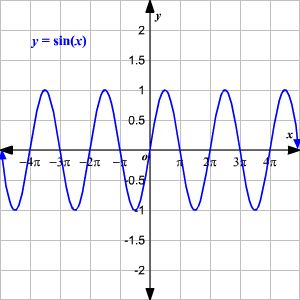Note that the domain of the function is the whole real line, while the range is $-1\le y\le 1$ .

The period of $f\left(x\right)=\mathrm{sin}\left(x\right)$ is $\text{2}\pi$ . That is, the shape of the curve repeats every $\text{2}\pi$ -